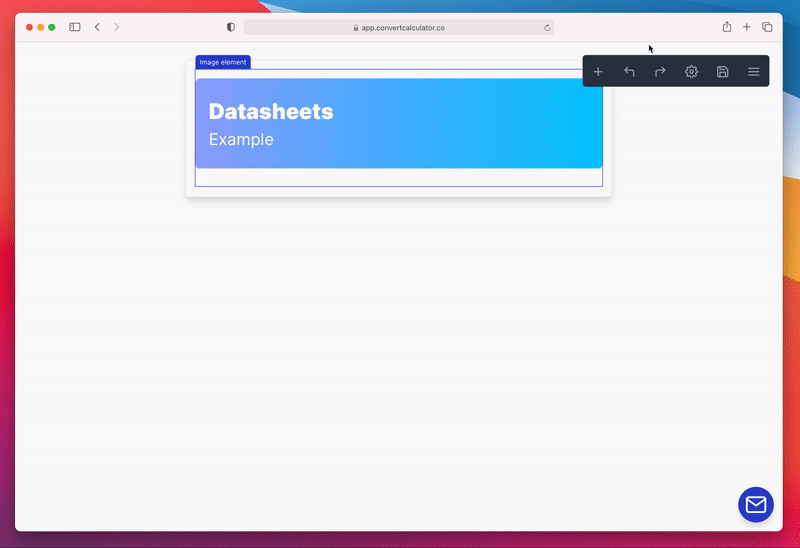# Using Datasheets

Learn how to use datasheets for calculations that depend on large amounts of data.

Sometimes you need to calculate something that can easily be put in a calculation. But sometimes, the outcome of a formula is based on larger datasets. Don't worry, though. For these use cases, we've also got you covered!

# How do Datasheets work?

Datasheets are similar to Excel-sheets and Google-sheets. The difference is that datasheets are simplified by design. You can create a datasheet by expanding the formula editor and clicking on the "Add datasheet" button.For the datasheet itself, you can copy/paste your data or add values manually. When you have setup your datasheet, you can use the complete datasheet (matrix) or specific columns in your calculations.

# How to use Datasheets?

The strongest use case for datasheets is to find an item in a dataset. This use case can be facilitated with multiple functions (INDEX, VLOOKUP, etc.). A simple example of retrieving an item in a datasheet would look like this:

`FS`INDEX(DA_A, 3)``

The `INDEX-function` will pick the 4th item (3 + 1, since datasheets start from 0) from column A in Datasheet A, which is referenced as `DA`.

For example Say your result depends on two inputs, in this example two option questions. For this, you can build a datasheet, with one question representing the x-axis (column numbers) and the one question the y-axis (row numbers). You can do this with the INDEX-function.Be aware that the INDEX starts from 0. When either the row indicator or the column indicator is 0, the formula will return the row/column label.

A second strong use case for datasheets is when you want to find a value that is matching one or multiple conditions.

`FS`FINDIFS(DA_C, DA_A, 1, DA_B, 2)``
Or
`FS`FINDIFS(DA_C, DA_A, QA, DA_B, QB)``
The `FINDIFS-function` finds the value in column C of datasheet A `DA` on the row where column A matches the static value 1 or the value returned by QA and column B matches 2/QB. This is well explained in the example below.## What functions can be used with datasheets?

These popular functions can be used with datasheets:

Note: there are other functions that accept columns and matrices as input. The functions above are the most common ones.

# Example

Below is an example to show how to use a datasheet properly. Let's assume we have the following datasheet (`DA`):

ABC
1150
12100
21125
22150

Column A stands for bedrooms, column B bathrooms, and column C is the price of cleaning. You have two questions (`QA: Bedrooms`, `QB: Bathrooms`) and want to calculate the cost of cleaning. Basically, you want to find the item in column C under conditions A and B. So 1 bedroom and 2 bathrooms would be \$100, and 2 bedrooms and 1 bathroom would be \$125.

The formula that finds the proper value is the following:

`FS`FINDIFS(DA_C, DA_A, QA, DA_B, QB)``

## Related articles